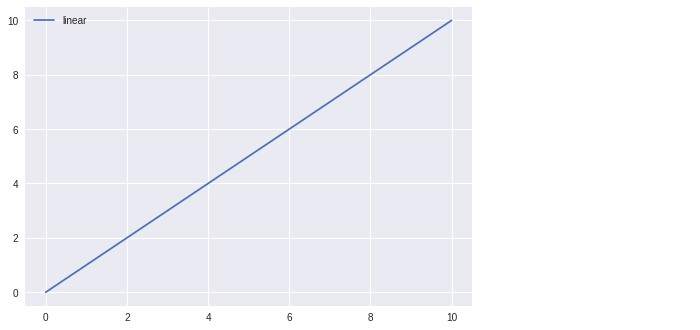# Top Python Machine Learning Libraries

## Introduction

As the name implies, machine learning is the practice of creating computer algorithms that can learn from various types of data. In a broader definition, Arthur Samuel states that "machine learning is the scientific field which offers computers the power to learn and without being expressly taught." They are frequently used to deal with a variety of everyday difficulties.

The algorithms, mathematical equations, and statistical calculations used in the past to perform Machine Learning tasks were all manually coded. As a result, the procedure was time-consuming, challenging, and ineffective. But because of a number of libraries, frameworks, and modules, it is now a lot simpler and more efficient than it was in the past. Due in part to its vast library collection, Python has replaced a number of other languages as one of the most popular ones for this activity right now. In this article we will explore Python libraries that are deployed in machine learning:

• NumPy

• Matplotlib

• TensorFlow

• Keras

• Theano

### NumPy

A well-known Python library called NumPy is used to compute enormous multidimensional arrays including matrices using a wide range of complex mathematical operations. Particularly helpful are its talents in linear algebra, the Fourier transform, and random numbers. NumPy is used internally by high-end libraries like TensorFlow to manipulate tensors. A quick computing toolkit called NumPy is capable of performing a wide range of operations, from simple algebra through Fourier transforms, random simulation, and shape manipulation.

import numpy as np
m = np.array([[2, 3], [5, 4]])
n = np.array([[2, 7], [4, 8]])
c = np.array([2, 12])
d = np.array([10, 13])
print (np.dot(c, d), "\n")
print (np.dot(m, c), "\n")
print (np.dot(m, n))


## Output

176
[40 58]
[[16 38]
[26 67]]


### Matplotlib

Matplotlib is a well-liked Python data visualization package. It provides a range of graphs & plots, such as histograms, error graphs, bar charts, etc., for displaying data. Before forwarding the data to data processing and machine learning training, it is helpful to visualize the data using the Matplotlib module in Python.

Matplotlib is an excellent Python visualization package for 2D array displays. To handle the larger SciPy stack, a multi-platform data visualization toolkit called Matplotlib was developed and is dependent on NumPy arrays. John Hunter introduced it for the first time in 2002.

One of the visualization's main benefits is that it makes it possible for us to view enormous amounts of data in straightforward formats. In Matplotlib, there are many different types of plots, such as line, bar, scatter, and histograms.

import matplotlib.pyplot as plt1
import numpy as np
m = np.linspace(0, 15, 95)
plt1.plot(m, m, label ='linear')
plt1.legend()
plt1.show()


## Output### TensorFlow

The Google Brain team at Google created TensorFlow, a very well-liked open-source toolkit for high-performing numerical calculation. TensorFlow is indeed a framework that allows the definition and execution of tensor-based calculations, as the name would imply. Deep neural networks which can be utilized to create a variety of AI applications can be trained and operated by it. In the area of machine learning research and application, TensorFlow is frequently used.

Although machine learning is a challenging area, it is now more simple to put machine-learning models into practice. This is due to the ease with which machine learning frameworks, such as Google's TensorFlow, make it possible to collect data, train models, produce predictions, and enhance future results.

The Google Brain team created the open-source TensorFlow framework in 2015, which may be used for large-scale machine learning as well as numerical computation. TensorFlow makes a number of data mining and machine learning models and approaches (often referred to as neural networks) practical. It provides a simple front-end API for creating Python or JavaScript programmes, which are subsequently run in high-performance C++.

### Keras

It comes with a number of integrated methods for collecting, integrating, and filtering data.

Theano, CNTK, or TensorFlow can all use this high-level neural network API. The CPU and GPU may both function without any problems. For ML beginners, Keras makes designing and building a neural network relatively straightforward. The ease and speed with which Keras facilitates prototyping is one of its best characteristics.

On Theano, TensorFlow, or CNTK, Keras, an open-source high-level neural network framework, can function. It was produced by Google developer Francois Chollet. to make deep neural network experimentation more quickly possible.

It is made consumer-friendly, modular, and extendable. Convolutional networks can be used alone.

It can't handle low-level computations, thus it utilizes the Backend library to resolve them. It may be run on TensorFlow, CNTK, or Theano thanks to the backend library, which acts as a high-level API wrapper for low-level API once more.

### Theano

We are all aware that math and statistics make up the majority of machine learning. A well-liked Python library called Theano is used to effectively design, assess, and optimize mathematical equations using multi-dimensional arrays. It is achieved by maximizing CPU and GPU use. It is an essential Python deep learning toolkit that you can use to build deep learning models directly or through wrapper libraries that make the process simpler.

## Conclusion

We gained knowledge of the top Python libraries for machine learning from this article. Each library has advantages and disadvantages. Before choosing a library for machine learning, these factors should be considered, and after training and evaluating the models, the accuracy of the models should be checked in order to choose the best model in the finest library to complete the assignment.